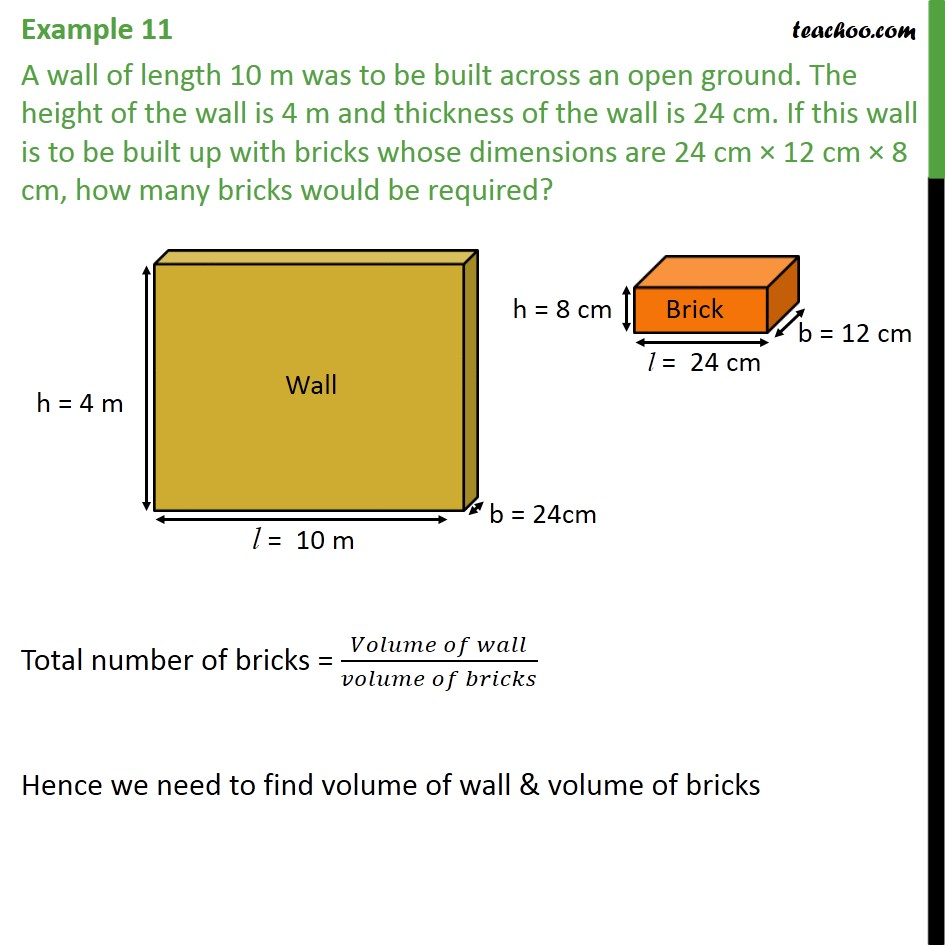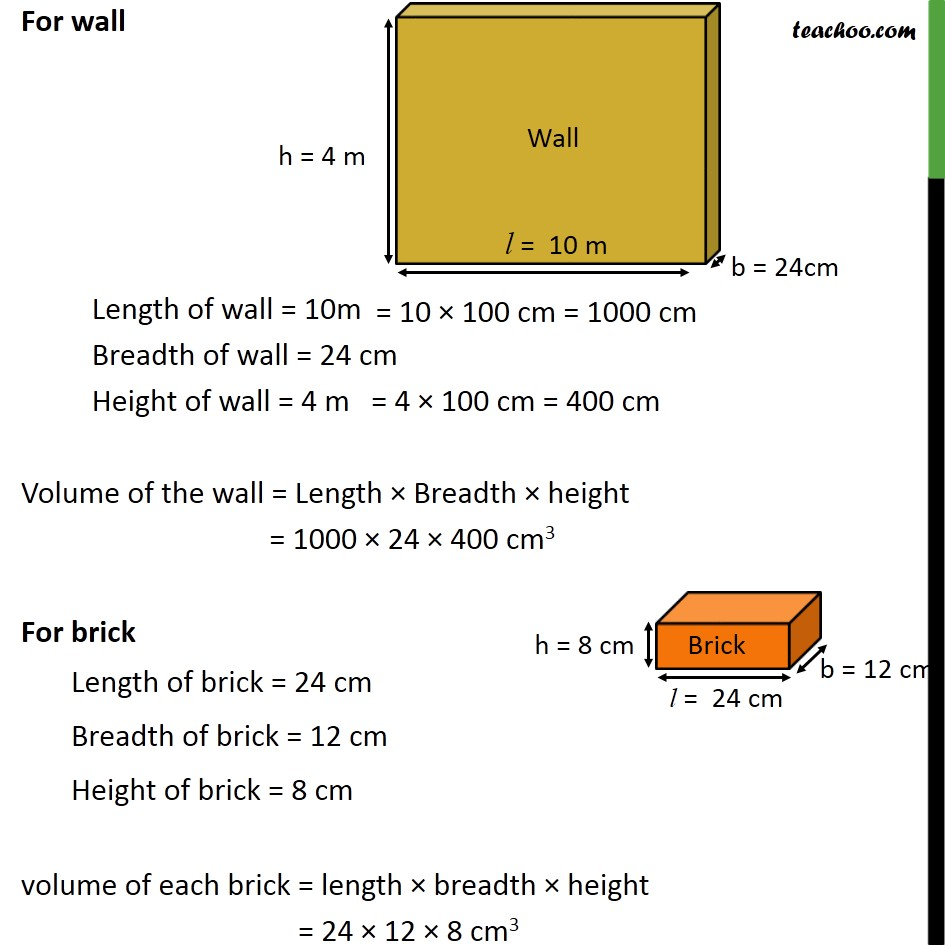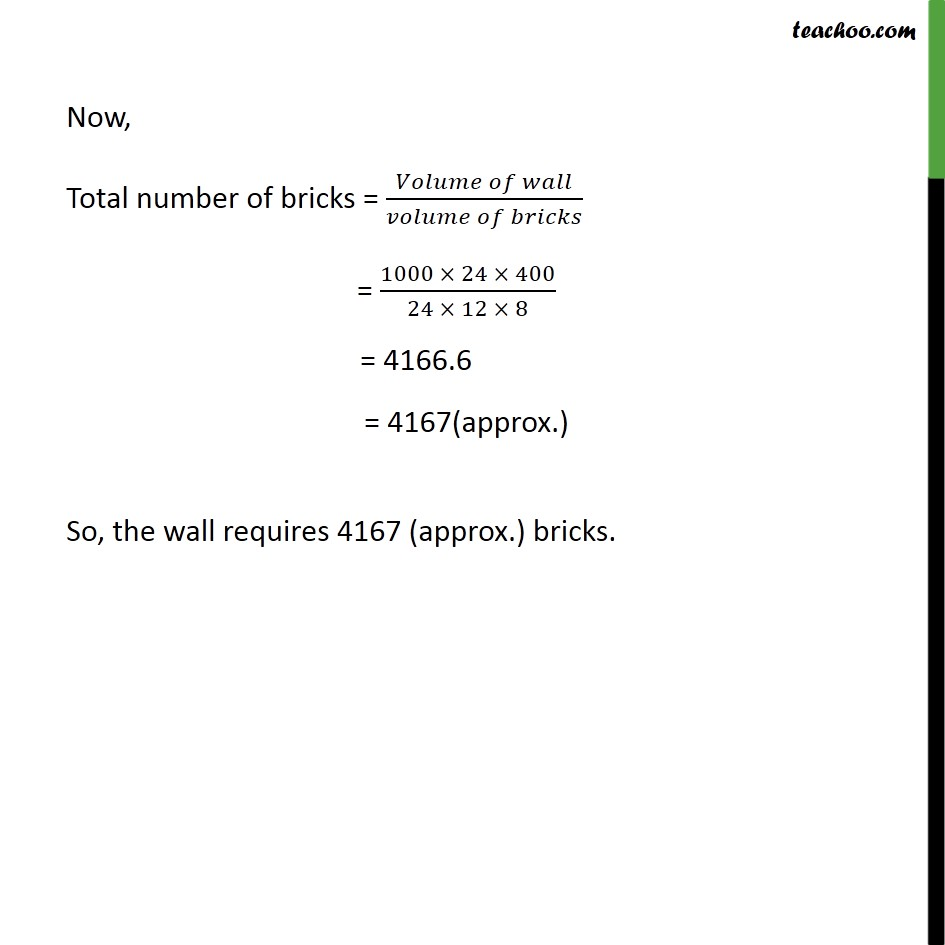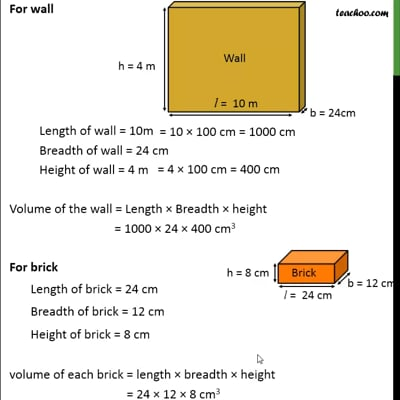Volume Of Cube/Cuboid

Chapter 13 Class 9 Surface Areas and Volumes
Concept wiseThis video is only available for Teachoo black users

Introducing your new favourite teacher - Teachoo Black, at only ₹83 per month

### Transcript

Example 11 A wall of length 10 m was to be built across an open ground. The height of the wall is 4 m and thickness of the wall is 24 cm. If this wall is to be built up with bricks whose dimensions are 24 cm × 12 cm × 8 cm, how many bricks would be required? Total number of bricks = (𝑉𝑜𝑙𝑢𝑚𝑒 𝑜𝑓 𝑤𝑎𝑙𝑙)/(𝑣𝑜𝑙𝑢𝑚𝑒 𝑜𝑓 𝑏𝑟𝑖𝑐𝑘𝑠) Hence we need to find volume of wall & volume of bricks For wall Length of wall = 10m Breadth of wall = 24 cm Height of wall = 4 m Volume of the wall = Length × Breadth × height = 1000 × 24 × 400 cm3 For brick Length of brick = 24 cm Breadth of brick = 12 cm Height of brick = 8 cm volume of each brick = length × breadth × height = 24 × 12 × 8 cm3 Now, Total number of bricks = (𝑉𝑜𝑙𝑢𝑚𝑒 𝑜𝑓 𝑤𝑎𝑙𝑙)/(𝑣𝑜𝑙𝑢𝑚𝑒 𝑜𝑓 𝑏𝑟𝑖𝑐𝑘𝑠) = (1000 × 24 × 400)/(24 × 12 × 8) = 4166.6 = 4167(approx.) So, the wall requires 4167 (approx.) bricks.